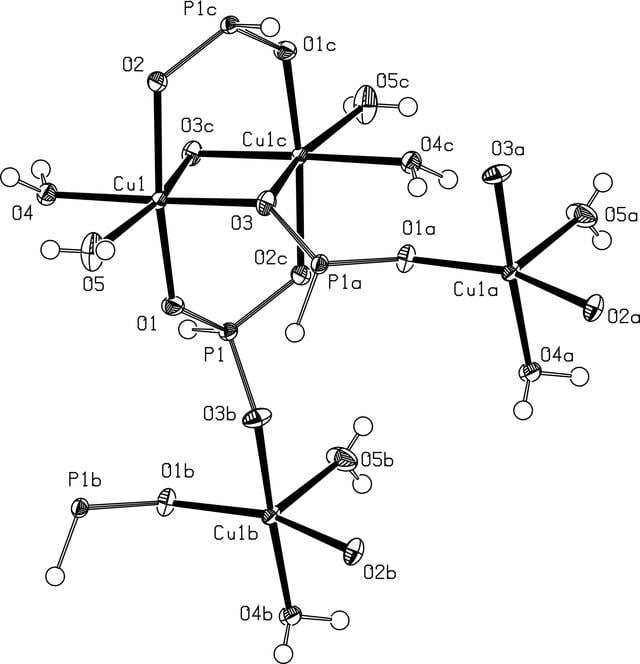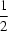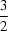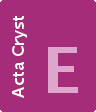disable zoom     view article Figure 1 A section of the structure of (I) showing the centrosymmetric SBU with the edge-sharing distorted CuO6 octa­hedra. Displacement ellipsoids are drawn at the 50% probability level. Atoms labelled a, b, c are symmetry-related. Symmetry codes: (a = − x, −+ y,− z; (b = − x,+ y,− z; (c = − x, − y, 1 − z).CRYSTALLOGRAPHICCOMMUNICATIONS
ISSN: 2056-9890